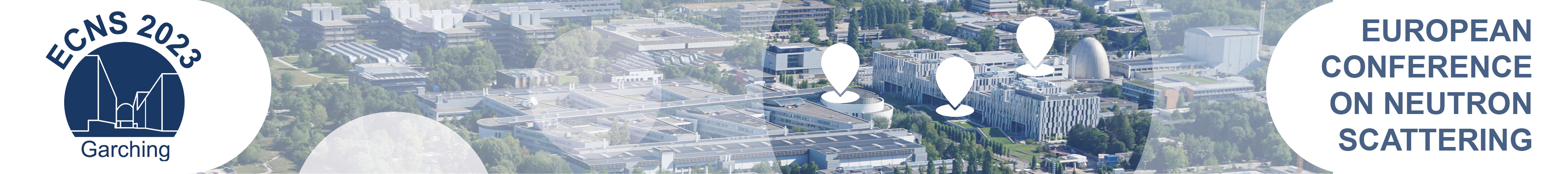#European Conference on Neutron Scattering 2023

Mar 20 – 23, 2023
Campus Garching
Europe/Berlin timezone

## Magnetic excitations in the zigzag-chain compound KCu$_4$P$_3$O$_{12}$

Mar 20, 2023, 4:00 PM
2h
Yards 4 - 6 (Fakultät für Maschinenwesen)

### Yards 4 - 6

#### Fakultät für Maschinenwesen

Board: MO-147
Poster Magnetism, Superconductivity, Topological Systems, Magnetic Thin Films an other electronic phenomena

### Speaker

Masashi Matsuo (National Institute for Materials Science)

### Description

We report on the results of inelastic neutron scattering (INS) of KCu$_4$P$_3$O$_{12}$. Cu$^{2+}$ ions carry $S = 1/2$ spins. Four types of nearest-neighbor exchange interactions form the eight-spin zigzag-chain ($J_1 - J_2 - J_3 - J_4 - J_3 - J_2 - J_1$ chain). We evaluated the exchange interactions as $J_1 = -8.5$ meV (antiferromagnetic), $J_2 = -2.7$ meV, $J_3 = -3.9$ meV, and $J_4 = 6.2$ meV (ferromagnetic) from magnetic susceptibility and magnetization curves at several temperatures using a data-driven technique based on machine learning proposed by Tamura and Hukushima. In the eight-spin zigzag-chain with the values of the exchange interactions, the ground state (GS) is the spin-singlet state. The first-triplet, second-triplet, third-quintet, and fourth-triplet excited states (1ES, 2ES, 3ES, and 4ES, respectively) are located at 2.9, 4.2, 6.7, and 8.5 meV, respectively.
We performed INS experiments on KCu$_4$P$_3$O$_{12}$ powder using the HRC spectrometer at J-PARC (Proposal ID 2020B0026). We used incident neutrons with the energy 15.3 and 25.4 meV. Excitations at 5.5 K are most apparent at around $\omega =3$ meV and $Q = 0.7$ Å$^{-1}$. The 3 meV excitation exists in a wide $Q$ range, indicating cluster excitation, and corresponds to the excitation from GS to 1ES (2.9 meV). Excitations are seen up to about 6 meV, indicating the existence of the excitation from GS to 2ES (4.2 meV). We can see weak excitations around 9 meV, corresponding to the excitation from GS to 4ES (8.5 meV). Probably, we also observed the excitation from 1ES to 3ES (3.8 meV) at high-temperature data. As a result, the calculated energies of 1ES, 2ES, 3ES, and 4ES from GS are consistent with the experimental results. We will discuss the $Q$ dependence of the INS intensity.

### Primary author

Masashi Matsuo (National Institute for Materials Science)

### Co-authors

Prof. Koji Hukushima (The University of Tokyo) Dr Ryo Tamura (National Institute for Materials Science) Dr Shinichiro Asai (The University of Tokyo) Prof. Takatsugu Masuda (The University of Tokyo)

### Presentation materials

There are no materials yet.